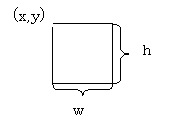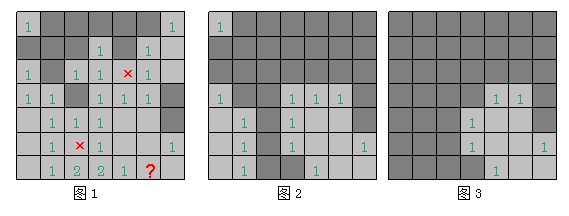# wxWidgets开发简单扫雷游戏程序之程序分析－《跟我学wxWidgets开发》系列教程

wxWidgets开发简单扫雷游戏程序之程序分析

5.2程序分析

void Init();                                       //游戏场景数据初始化
void DrawGrid();                             //绘制游戏场景
void CreateMinesTips(int pos);      //生成个数提示数据
int HitTest(wxPoint &point);          //鼠标事件区域检测
void HitCheck(int pos);                   //鼠标点击单元格时是否是地雷的判定
void FindSpaceNearby(int pos);     //找出相邻的安全空白区域
void ShowTipsAround(int pos);      //鼠标右击提示单元时显示周围可能的雷区
bool EdgeCheck(int pos,int newpos);//场景边缘检测
void OpenAll();                                //打开并显示所有单元

void SetLevel(int level=0);              //设置游戏等级
int GetLevel();                                 //获取当前游戏等级

void OnQuit(wxCommandEvent& event);                               //退出菜单事件
void OnPaint(wxPaintEvent& event);                                     //窗口重绘事件
void OnLeftDown(wxMouseEvent& event);                                          //鼠标左击按下事件
void OnRightDown(wxMouseEvent& event);                         //鼠标右击按下事件
void OnRightUp(wxMouseEvent& event);                              //鼠标右击松开事件

5.2.1 游戏对象数据结构

struct Mine{
int flag;   //>=10:mines,0<flag<10:mine number around,0:space
int statu;  //0:closed,1:opened,2:sweeped
bool tips;
};

1) flag——用来标识单元格里的内容
Flag>=10时，内容为地雷
0<Flag<10时，内容为地雷旁边的个数提示（因为周围最大地雷数为8，所以定此范围）
Flag=0时，内容为空白（里面啥都没有）
2)statu——用来标识单元格的三种状态
Statu=0时，单元格没有被点开的状态
Statu=1时，单元格被点开的状态
Statu=2时，单元格没被点开时被右击标记为地雷的状态
3)tips——用来记录周围的地雷个数

5.2.2 通过WX_DEFINE_ARRAY实现结构体数组

WX_DEFINE_ARRAY(对象类型或指针,容器类型名);
WX_DEFINE_ARRAY(Mine *, ArrayOfMines);

ArrayOfMines m_arrMines;

5.2.3 通过wxClientDC进行绘图

1）比如说wxClientDC(this)，就是把当前窗体wxFrame或wxDialog的客户区作为整个绘图区域；
2）又比如说我们的程序里有一个wxPanel对象panel1，wxClientDC(panel1)就意味着只在这个panel1中进行绘图；

5.2.4 HitTest鼠标事件坐标检测START FUNCTION
IF x0>x AND x0<(x+w) THEN
IF y0>y AND y0<(y+h) THEN
RETURN true;
END IF
END IF
RETURN false;
END FUNCTION

※思考：程序里的代码和伪代码逻辑上有一点小差别，你发现了吗，想一想为什么呢？

※5.2.5 相邻空白单元寻找算法//参数pos即当前单元在数组里的下标值
void minesweepingFrame::FindSpaceNearby(int pos)
{
if(!m_bGameStatu)   return;        //判断是否正在游戏状态
if(pos<0 || pos>m_nRectSize) return;

int newpos={pos-m_nRectUnit,pos-1,pos+1,pos+m_nRectUnit};
for(int i=0;i<4;i++)
{
if(newpos[i]>=0 && newpos[i]<m_nRectSize)
{
//when in the edge
if(EdgeCheck(pos,newpos[i]))   continue;

if(m_arrMines.Item(newpos[i])->flag==0 && m_arrMines.Item(newpos[i])->statu==0)
{
m_arrMines.Item(newpos[i])->statu=1;
FindSpaceNearby(newpos[i]);
}

//show simple 1
if(m_arrMines.Item(newpos[i])->flag==1 && m_arrMines.Item(newpos[i])->statu==0)
{
m_arrMines.Item(newpos[i])->statu=1;
}
}
}
}

bool minesweepingFrame::EdgeCheck(int pos,int newpos)
{
//m_nRectUnit可以理解为游戏场景中每一行的单元格数量
if(pos%m_nRectUnit==0)   //left edge
{
if(newpos==pos-(m_nRectUnit+1) || newpos==pos-1 || newpos==pos+(m_nRectUnit-1))  return true;
}
else if((pos+1)%m_nRectUnit==0)   //right edge
{
if(newpos==pos-(m_nRectUnit-1) || newpos==pos+1 || newpos==pos+(m_nRectUnit+1))  return true;
}

if(pos-m_nRectUnit<0)    //up edge
{
if(newpos==pos-(m_nRectUnit+1) || newpos==pos-m_nRectUnit || newpos==pos-(m_nRectUnit-1))  return true;
}

if(pos+m_nRectUnit>=m_nRectSize) //down edge
{
if(newpos==pos+(m_nRectUnit+1) || newpos==pos+m_nRectUnit || newpos==pos+(m_nRectUnit-1))  return true;
}

return false;
}

1